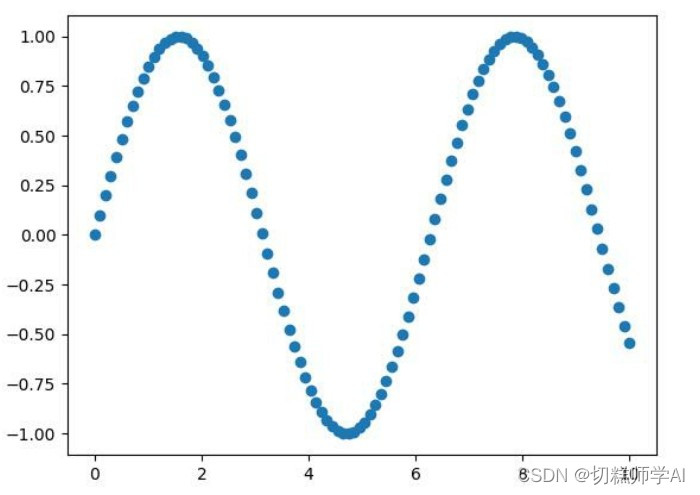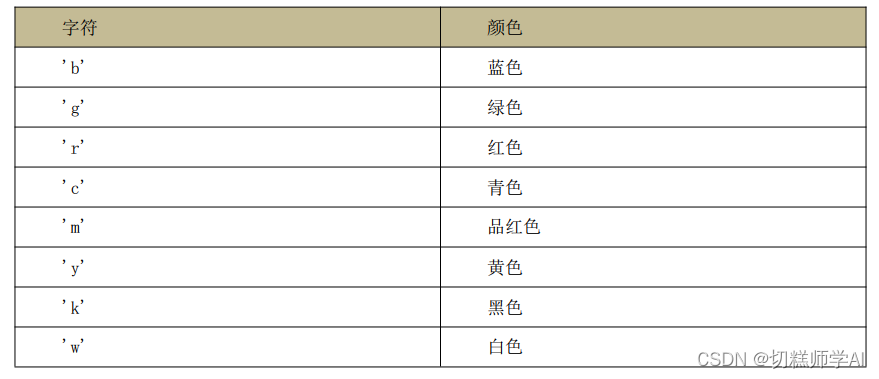﻿ Python Matplotlib数据可视化模块使用详解_python_脚本之家
python# Python Matplotlib数据可视化模块使用详解

matplotlib是基建立在python之上，适用于创建静态，动画和交互式可视化，通常与数据分析模块pandas搭配使用，用于数据的分析和展示，适用于主流的操作系统，如Linux、Win、Mac

• 了解什么是 Matplotlib
• 掌握如何用 Matplotlib 绘制各种图形（柱状图、饼状图、直方图等）
• 掌握如何定制图形的颜色和样式
• 掌握如何用 Matplotlib 绘制三维图

## 前言

Matplotlib 是一个 Python 的 2D 绘图库。通过 Matplotlib，开发者可以仅需要几行代码，便可以生成绘图，直方图，功率谱，条形图，错误图，散点图等。学习 Matplotlib，可让数据可视化，更直观的真实给用户。使数据更加客观、更具有说服力。 Matplotlib 是 Python的库，又是开发中常用的库。

## 1 matplotlib 开发环境搭建

pip install matplotlib

`import matplotlib.pyplot as plt`

## 2 绘制基础

pyplot 基本方法的使用如下。### 2.1 绘制直线

• 导入模块 pyplot，并给它指定别名 plt，以免反复输入 pyplot。在模块 pyplot中包含很多用于生产图表的函数。
• 将绘制的直线坐标传递给函数 plot()。
• 通过函数 plt.show()打开 Matplotlib 查看器，显示绘制的图形。

【示例 1】使用 matplotlib 根据两点绘制一条线

```import matplotlib.pyplot as plt
#将(0,1)点和(2,4)连起来
plt.plot([0,2],[1,4])
plt.show()
```### 2.2 绘制折线

【示例 2】使用 matplotlib 绘制折线图

```import matplotlib.pyplot as plt
x=[1,2,3,4,5]
squares=[1,4,9,16,25]
plt.plot(x,squares)
plt.show()
```### 2.3 设置标签文字和线条粗细

【示例 3】使用 matplotlib 绘制折线图并设置样

```import matplotlib.pyplot as plt
datas=[1,2,3,4,5]
squares=[1,4,9,16,25]
plt.plot(datas,squares,linewidth=5)
#设置线条宽度#设置图标标题，并在坐标轴上添加标签plt.title('Numbers',fontsize=24)
plt.xlabel('datas',fontsize=14)
plt.ylabel('squares',fontsize=14)
plt.show()
```Matplotlib 默认情况不支持中文，可以使用以下简单的方法来解决：

`plt.rcParams['font.sans-serif']=['SimHei'] #用来正常显示中文标签`

【示例 4】解决标签、标题中的中文问题

```import matplotlib.pyplot as plt
datas=[1,2,3,4,5]
squares=[1,4,9,16,25]
plt.plot(datas,squares,linewidth=5)
#设置线条宽度#设置中文乱码问题
plt.rcParams['font.sans-serif'] = ['SimHei']
#设置图标标题，并在坐标轴上添加标签
plt.title('标题设置',fontsize=24)
plt.xlabel('x 轴',fontsize=14)
plt.ylabel('y 轴',fontsize=14)
plt.show()
```### 2.4 绘制一元二次方程的曲线 y=x^2

Matplotlib 有很多函数用于绘制各种图形，其中 plot 函数用于曲线，需要将 200 个点的x 坐标和 Y 坐标分别以序列的形式传入 plot 函数，然后调用 show 函数显示绘制的图形。一元二次方程的曲线。

【示例 5】使用 matplotlib 绘制一元二次方程曲线

```import matplotlib.pyplot as plt
#200 个点的 x 坐标
x=range(-100,100)
#生成 y 点的坐标
y=[i**2 for i in x ]
#绘制一元二次曲线
plt.plot(x,y)
#调用 savefig 将一元二次曲线保存为 result.jpg
#plt.savefig('result.jpg') #如果直接写成 plt.savefig('cos')  会生成 cos.png
plt.show()
```### 2.5 绘制正弦曲线和余弦曲线

【示例 6】使用 matplotlib 绘制正弦曲线和余弦曲线

```import matplotlib.pyplot as plt
import numpy as np
#生成 x 的坐标（0-10 的 100 个等差数列）
x=np.linspace(0,10,100)
sin_y=np.sin(x)
#绘制正弦曲线
plt.plot(x,sin_y)
#绘制余弦曲线
cos_y=np.cos(x)
plt.plot(x,cos_y)
plt.show()
``````import matplotlib.pyplot as plt
import numpy as np
#将画布分为区域，将图画到画布的指定区域
x=np.linspace(1,10,100)
#将画布分为 2 行 2 列，将图画到画布的 1 区域
plt.subplot(2,2,1)
plt.plot(x,np.sin(x))
plt.subplot(2,2,3)
plt.plot(x,np.cos(x))
plt.show()
```## 3 绘制散点图

【示例 8】使用 matplotlib 绘制 sin()函数的散点图

```import matplotlib.pyplot as plt
import numpy as np
#画散点图
x=np.linspace(0,10,100)
#生成 0 到 10 中 100 个等差数
plt.scatter(x,np.sin(x))
plt.show()
```【示例 9】绘制 10 种大小 100 种颜色的散点图

```import matplotlib.pyplot as plt
import numpy as np
#  画 10 种大小， 100 种颜色的散点图
np.random.seed(0)
x=np.random.rand(100)
y=np.random.rand(100)
colors=np.random.rand(100)
size=np.random.rand(100)*1000
plt.scatter(x,y,c=colors,s=size,alpha=0.7)
plt.show()
```【示例 10】绘制不同种类不同颜色的线

```import matplotlib.pyplot as plt
import numpy as np
#不同种类不同颜色的线
x=np.linspace(0,10,100)
plt.plot(x,x+0,'-g')	#实线  绿色
plt.plot(x,x+1,'--c')	#虚线 浅蓝色
plt.plot(x,x+2,'-.k')	#点划线 黑色
plt.plot(x,x+3,'-r')	#实线  红色
plt.plot(x,x+4,'o')	#点   默认是蓝色
plt.plot(x,x+5,'x')	#叉叉  默认是蓝色
plt.plot(x,x+6,'d')	#砖石  红色
plt.show()
```【示例 11】添加图例

```#不同种类不同颜色的线并添加图例
x=np.linspace(0,10,100)
plt.plot(x,x+0,'-g',label='-g')	#实线  绿色
plt.plot(x,x+1,'--c',label='--c')	#虚线 浅蓝色
plt.plot(x,x+2,'-.k',label='-.k')	#点划线 黑色
plt.plot(x,x+3,'-r',label='-r')	#实线  红色
plt.plot(x,x+4,'o',label='o')	#点   默认是蓝色
plt.plot(x,x+5,'x',label='x')	#叉叉  默认是蓝色
plt.plot(x,x+6,'dr',label='dr')	#砖石  红色
#添加图例右下角 lower right	左上角 upper left  边框  透明度  阴影  边框宽度
plt.show()
```## 4 绘制柱状图

【示例 12】使用 bar()绘制柱状图，并设置柱的宽度

```import matplotlib.pyplot as plt
import numpy as np
x=[1980,1985,1990,1995]
x_labels=['1980 年','1985 年','1990 年','1995 年']
y=[1000,3000,4000,5000]
plt.bar(x,y,width=3)
plt.rcParams['font.sans-serif']=['SimHei'] #用来正常显示中文标签
plt.xticks(x,x_labels)
plt.xlabel('年份')
plt.ylabel('销量')
plt.title('根据年份销量对比图')
plt.show()
```【示例 13】使用 bar()和 barh()函数绘制柱状图

```import matplotlib.pyplot as plt
import numpy as np
np.random.seed(0)
x=np.arange(5)
y=np.random.randint(-5,5,5)
print(x,y)
# 将画布分隔成一行两列
plt.subplot(1,2,1)
#在第一列中画图
v_bar=plt.bar(x,y)
#在第一列的画布中 0 位置画一条蓝线
plt.axhline(0,color='blue',linewidth=2)
plt.subplot(1,2,2)
#barh 将 y 和 x 轴对换 竖着方向为 x 轴
h_bar=plt.barh(x,y,color='red')
#在第二列的画布中 0 位置处画蓝色的线
plt.axvline(0,color='red',linewidth=2)
plt.show()
```【示例 14】对柱状图的部分柱状设置颜色

```import matplotlib.pyplot as plt
import numpy as np
np.random.seed(0)
x=np.arange(5)
y=np.random.randint(-5,5,5)
v_bar=plt.bar(x,y,color='lightblue')
for bar,height in zip(v_bar,y):
if height<0:
bar.set(edgecolor='darkred',color='lightgreen',linewidth=3)
plt.show()
```【示例 15】使用 bar()绘制三天中三部电影的票房变化

```import matplotlib.pyplot as plt
import numpy as np
#三天中三部电影的票房变化
real_names=['千与千寻','玩具总动员 4','黑衣人：全球追缉']
real_num1=[5453,7548,6543]
real_num2=[1840,4013,3421]
real_num3=[1080,1673,2342]
#生成 x	第 1 天   第 2 天   第 3 天
x=np.arange(len(real_names))
x_label=['第{}天'.format(i+1) for i in range(len(real_names))]
#绘制柱状图#设置柱的宽度
width=0.3
plt.bar(x,real_num1,color='g',width=width,label=real_names)
plt.bar([i+width for i in x],real_num2,color='b',width=width,label=real_names)
plt.bar([i+2*width for i in x],real_num3,color='r',width=width,label=real_names)
plt.rcParams['font.sans-serif']=['SimHei'] #用来正常显示中文标签
#修改 x 坐标
plt.xticks([i+width for i in x],x_label)
#添加图例plt.legend() #添加标题
plt.title('3 天的票房数')
plt.show()
```## 5 绘制饼状图

pie 函数可以绘制饼状图，饼图主要是用来呈现比例的。只要传入比例数据即可。

【示例 16】绘制饼状图

```#导入模块
import matplotlib.pyplot as plt
import numpy as np
#准备男、女的人数及比例
man=71351
woman=68187
man_perc=man/(woman+man)
woman_perc=woman/(woman+man) #添加名称
labels=['男','女'] #添加颜色
colors=['blue','red'] #绘制饼状图  pie
plt.rcParams['font.sans-serif']=['SimHei'] #用来正常显示中文标签
# labels  名称 colors：颜色，explode=分裂  autopct 显示百分比
paches,texts,autotexts=plt.pie([man_perc,woman_perc],labels=labels,colors=colors,explode=(0,0.05),autopct='%0.1f%%')
#设置饼状图中的字体颜色
for text in autotexts:
text.set_color('white')
#设置字体大小
for text in texts+autotexts:
text.set_fontsize(20)
plt.show()
```## 6 绘制直方图

【示例 17】绘制直方图

```import numpy as np
import matplotlib.pyplot as plt
#频次直方图，均匀分布
#正太分布
x=np.random.randn(1000)
#画正太分布图
# plt.hist(x)
plt.hist(x,bins=100) #装箱的操作，将 10 个柱装到一起及修改柱的宽度
plt.show()
```【示例 18】同一画布绘制三个直方图

```import numpy as np
import matplotlib.pyplot as plt
#几个直方图画到一个画布中,第一个参数期望  第二个均值
x1=np.random.normal(0,0.8,1000)
x2=np.random.normal(-2,1,1000)
x3=np.random.normal(3,2,1000)
#参数分别是 bins：装箱，alpha：透明度
kwargs=dict(bins=100,alpha=0.4)
plt.hist(x1,**kwargs)
plt.hist(x2,**kwargs)
plt.hist(x3,**kwargs)
plt.show()
```## 7 绘制等高线图

【 示例 19 】使用matplotlib 绘制等高线图

```#导入模块
import matplotlib.pyplot as plt
import numpy as np
x=np.linspace(-10,10,100)
y=np.linspace(-10,10,100)
#计算 x 和 y 的相交点 a
X,Y=np.meshgrid(x,y)
# 计算 Z 的坐标
Z=np.sqrt(X**2+Y**2)
plt.contourf(X,Y,Z)
plt.contour(X,Y,Z)
# 颜色越深表示值越小，中间的黑色表示 z=0.
plt.show()
```## 8 绘制三维图

【示例 20】使用 Matplotlib 绘制三维图

```import matplotlib.pyplot as plt
#导入 3D 包
from mpl_toolkits.mplot3d import Axes3D
#创建 X、Y、Z 坐标
X=[1,1,2,2]
Y=[3,4,4,3]
Z=[1,100,1,1]
fig = plt.figure()
#  创建了一个 Axes3D 的子图放到 figure 画布里面
ax = Axes3D(fig)
ax.plot_trisurf(X, Y, Z)
plt.show()
```